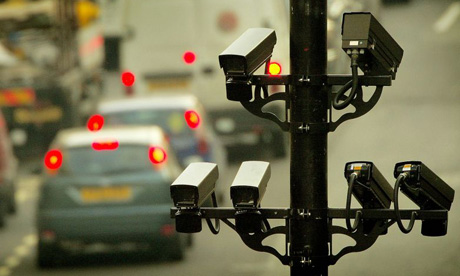# Storing the Images of Surveillance Cameras in Constant Space Using Infinite Geometric Series

I was recently in London – it’s a fantastic city and I loved my time there. However, I was completely baffled by the large amount of surveillance cameras in the city. Almost everywhere you go the Queen’s watching you.While I won’t go into a debate on whether or not surveillance is a good thing, I do want to discuss the sheer amount of data these cameras must pick up on a daily basis. I mean, several cameras on every street recording video non-stop – that must be super expensive in storage. Is there any way we can circumvent this?

## The Naïve Solution

An often used solution to the storage problem is to delete all images older than X days. This keeps the storage space needed constant and ensures that the most recent (and most relevant) photo information is readily available to check if needed. The most obvious disadvantage here is that the old images get deleted completely, making forensics difficult if the crime was committedso early that the camera has deleted all the relevant images. In this post we’ll detail an algorithm which keeps track of all images the surveillance camera has saved, yet accomplishes it in constant space.

# Storing the Images of Surveillance Cameras in Constant Space using Infinite Series

For the sake of this post, let’s assume the surveillance cameras take still photos every X minutes. The reason for this is that this algorithm probably doesn’t work well with existing video codecs (or at least, I wouldn’t know where to start). Now, obviously we can’t just store an indefinite amount of high-quality images on a drive with a limited storage space, so what we’re going to do is let the old images degrade over time. That is, the older the images is, the worse quality it has.

This makes sense – we assume that the most relevant images are the most recent images. After all, why would the police need to look at 3 year old surveillance photos of a random gas station? (There certainly could be incentive to look at old images, but we assume it’s the recent photos that we need to have in the best quality).

## The algorithm

We’ll let the images degrade with a constant factor$r \in [0, 1]$ for every constant amount of images. That is, the first$n$ images are saved in quality$Q = 1$ where$Q$ represents how large a percentage of the data we’re keeping for the image, so$Q=0.5$ means that we have reduced the space by 50%. The next$n$ images are saved in quality$Q = r$, and the next$n$ images after these are saved in quality$Q=r^2$.

We’ll call the first$n$ images for the priority 1 images, the next$n$ images for the priority 2 images etc. If we assume all images require a maximum disk space of$S$, then obviously the total disk space$D$ for all priority 1 images is$D_1 = n \cdot S$. Likewise, the total disk space for all priority 2 images is$D_2 = r \cdot n \cdot S$. And in general for priority$p$ images, we get:$D_p = r^{p-1} \cdot n \cdot S$

And for the first$k$ priorities we get the total disk space$T$:$T_k = D_1 + D_2 + D_3 + ... + D_k$

Which can be written using sigma-notation:$T_k = \displaystyle \sum_{i=1}^{k} D_i$

Expanding this, we get:$T_k = \displaystyle \sum_{i=1}^{k} r^{i-1} \cdot n \cdot S$

Switching our terms, we get:$T_k = n \cdot S \cdot \displaystyle \sum_{i=0}^k r^i$

To show that the total disk space is constant no matter how long we let our surveillance cameras run, we need to show that no matter how many priorities we sum up we’ll always remain bounded below some finite constant. That is, we look at the limit as we increase$k$:$T_\infty = n \cdot S \cdot \displaystyle \sum_{i=0}^\infty r^i$

And since this is a geometric series, we get the following expression:$T_\infty = \dfrac{n \cdot S}{1 - r}$

Which is finite for$n,S,r \in \mathbb{R}$ proving that the disk space required is constant with this approach.

## An example

Let’s say each surveillance camera has a total disk space of$T = 2 ~ \textrm{Gb}$ (2,147,483,648 bytes), and that each image is stored in the JPEG file format with size 480p (720 x 480). According to Wikipedia, a$Q=1$ image requires about 8.25 bits per color pixel, which means that each photo takes up$S = 348 ~ \textrm{Kb}$.

Assuming we take one image every minute from our surveillance cameras, and that we want the images to degrade on a daily basis (all images taken the same day are of the same quality), then$n = 1440$ images.

Finally, we can easily derive:$r = 1 - \dfrac{n \cdot S}{T}$

Which means that$r = 0.768$, which means that the images degrade roughly 23% in quality every day given this setup (obviously the parameters can vary, but this was just one example). Notice that this formula gives$r \notin [0,1]$ if$n \cdot S > T$. This makes sense because it implies that the priority 1 images themselves take up more disk space than the surveillance cameras (which is of course impossible).

I’m not sure how practical this would be to implement, but I found the thought interesting at least.